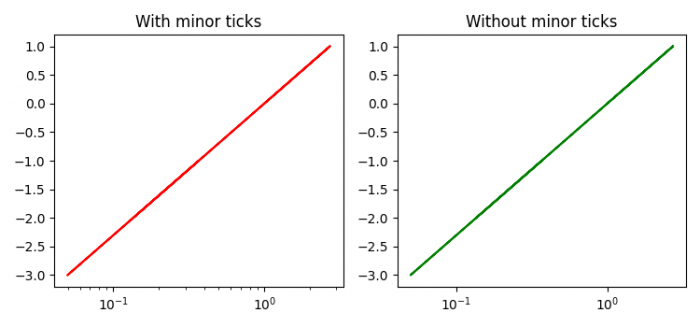# How to disable the minor ticks of a log-plot in Matplotlib?

To disable the minor ticks of a log plot in matplotlib, we can use minorticks_off() method.

## Steps

• Set the figure size and adjust the padding between and around the subplots.
• Create x and y data points using numpy.
• Add a subplot to the current figure, at index 1.
• Plot x and y data points with color=red.
• Make x-scale as log class by name.
• Set the title of the current plot.
• Add a subplot to the current figure, at index 2.
• Plot x and y data points with color=green.
• Make x-scale as log class by name.
• Turn off the minor ticks of the plot.
• Set the title of the plot as index 2.
• To display the figure, use show() method.

## Example

import numpy as np
from matplotlib import pyplot as plt
plt.rcParams["figure.figsize"] = [7.50, 3.50]
plt.rcParams["figure.autolayout"] = True
x = np.random.randint(-3, 3, 10)
y = np.exp(x)
plt.subplot(121)
plt.plot(y, x, c='red')
plt.xscale('log')
plt.title("With minor ticks")
plt.subplot(122)
plt.plot(y, x, c='green')
plt.xscale('log')
plt.minorticks_off()
plt.title("Without minor ticks")
plt.show()

## Output